# superconductivity

(redirected from superconduct)
Also found in: Dictionary, Thesaurus.

## superconductivity,

abnormally high electrical conductivity of certain substances. The phenomenon was discovered in 1911 by Heike Kamerlingh OnnesKamerlingh Onnes, Heike
, 1853–1926, Dutch physicist. He was, from 1882, professor of physics at the Univ. of Leiden. He made important studies of the properties of helium and, in attempting to solidify it, produced a temperature within one degree of absolute zero.
, who found that the resistance of mercury dropped suddenly to zero at a temperature of about 4.2°K;; he received (1913) the Nobel Prize for the discovery. For the next 75 years there followed a rather steady string of announcements of new materials that become superconducting near absolute zero. A major breakthrough occurred in 1986 when Karl Alexander MüllerMüller, Karl Alexander,
1927–, Swiss physicist, Ph.D. Swiss Federal Institute of Technology, 1958. In 1983, Müller and co-researcher Johannes Georg Bednorz discovered superconductivity in a ceramic fragment at temperatures much higher than had been previously
and J. Georg BednorzBednorz, Johannes Georg
, 1950–, German physicist. After earning his doctorate from the Swiss Federal Institute of Technology, he began (1982) work at the IBM Zürich Research Laboratory with Karl Alex Müller.
announced that they had discovered a new class of copper-oxide materials that become superconducting at temperatures exceeding 70°K;. The work of Müller and Bednorz, which earned them the Nobel Prize in Physics in 1987, precipitated a host of discoveries of other high-temperature cuprate superconductors that exhibit lossless electrical flow at temperatures up to nearly 140°K;. In 2008 Hideo Hosono and a Japanese team announced the discovery of a iron-arsenic high-temperature superconductor, and since then other such iron-based superconductors have been identified.

Classical superconductivity (superconductivity at temperatures near absolute zero) is displayed by some metals, including zinc, magnesium, lead, gray tin, aluminum, mercury, and cadmium. Other metals, such as molybdenum, may exhibit superconductivity after high purification. More than 50 elements are superconductive at temperatures near absolute; some, such as europium, only under extreme pressure as well. Alloys (e.g., two parts of gold to one part of bismuth) and such compounds as tungsten carbide and lead sulfide may also be superconductors.

Thin films of normal metals and superconductors that are brought into contact can form superconductive electronic devices, which replace transistors in some applications. An interesting aspect of the phenomenon is the continued flow of current in a superconducting circuit after the source of current has been shut off; for example, if a lead ring is immersed in liquid helium, an electric current that is induced magnetically will continue to flow after the removal of the magnetic field. Powerful electromagnets, which, once energized, retain magnetism virtually indefinitely, have been developed using several superconductors.

The 1972 Nobel Prize in Physics was awarded to J. Bardeen, L. Cooper, and S. Schrieffer for their theory (known as the BCS theory) of classical superconductors. This quantum-mechanical theory proposes that at very low temperatures electrons in an electric current move in pairs. Such pairing enables them to move through a crystal lattice without having their motion disrupted by collisions with the lattice. Several theories of high-temperature superconductors have been proposed, but none has been experimentally confirmed.

### Bibliography

See J. W. Lynn, ed., High-Temperature Superconductivity (1990).

## Superconductivity

A phenomenon occurring in many electrical conductors, in which the electrons responsible for conduction undergo a collective transition into an ordered state with many unique and remarkable properties. These include the vanishing of resistance to the flow of electric current, the appearance of a large diamagnetism and other unusual magnetic effects, substantial alteration of many thermal properties, and the occurrence of quantum effects otherwise observable only at the atomic and subatomic level.

Superconductivity was discovered by H. Kamerlingh Onnes in Leiden in 1911 while studying the temperature dependence of the electrical resistance of mercury within a few degrees of absolute zero. He observed that the resistance dropped sharply to an unmeasurably small value at a temperature of 4.2 K (-452°F). The temperature at which the transition occurs is called the transition or critical temperature, Tc. The vanishingly small resistance (very high conductivity) below Tc suggested the name given the phenomenon.

In 1933 W. Meissner and R. Ochsenfeld discovered that a metal cooled into the superconducting state in a moderate magnetic field expels the field from its interior. This discovery demonstrated that superconductivity involves more than simply very high or infinite electrical conductivity, remarkable as that alone is. See Meissner effect

In 1957, J. Bardeen, L. N. Cooper, and J. R. Schrieffer reported the first successful microscopic theory of superconductivity. The Bardeen-Cooper-Schrieffer (BCS) theory describes how the electrons in a conductor form the ordered superconducting state. The BCS theory still stands as the basic explanation of superconductivity, even though extensive theoretical work has embellished it.

There are a number of practical applications of superconductivity. Powerful superconducting electromagnets guide elementary particles in particle accelerators, and they also provide the magnetic field needed for magnetic resonance imaging. Ultrasensitive superconducting circuits are used in medical studies of the human heart and brain and for a wide variety of physical science experiments. A completely superconducting prototype computer has even been built. See Particle accelerator, Superconducting devices

#### Transition temperatures

It was realized from the start that practical applications of superconductivity could become much more widespread if a high-temperature superconductor, that is, one with a high Tc, could be found. For instance, the only practical way to cool superconductors with transition temperatures below 20 K (-424°F) is to use liquid helium, which boils at a temperature of 4.2 K (-452°F) and which is rather expensive. On the other hand, a superconductor with a transition temperature of 100 K (-280°F) could be cooled with liquid nitrogen, which boils at 77 K (-321°F) and which is roughly 500 times less expensive than liquid helium. Another advantage of a high-Tc material is that, since many of the other superconducting properties are proportional to Tc, such a material would have enhanced properties. In 1986 the discovery of transition temperatures possibly as high as 30 K (-406°F) was reported in a compound containing barium, lanthanum, copper, and oxygen. In 1987 a compound of yttrium, barium, copper, and oxygen was shown to be superconducting above 90 K (-298°F). In 1988 researchers showed that a bismuth, strontium, calcium, copper, and oxygen compound was superconducting below 110 K (-262°F), and transition temperatures as high as 135 K (-216°F) were found in a mercury, thallium, barium, calcium, copper, and oxygen compound.

#### Occurrence

Some 29 metallic elements are known to be superconductors in their normal form, and another 17 become superconducting under pressure or when prepared in the form of thin films. The number of known superconducting compounds and alloys runs into the thousands. Superconductivity is thus a rather common characteristic of metallic conductors. The phenomenon also spans an extremely large temperature range. Rhodium is the element with the lowest transition temperature (370 μK), while Hg0.2Tl0.8Ca2Ba2Cu3O is the compound with the highest (135 K or -216°F).

Despite the existence of a successful microscopic theory of superconductivity, there are no completely reliable rules for predicting whether a metal will be a superconductor. Certain trends and correlations are apparent among the known superconductors, however—some with obvious bases in the theory—and these provide empirical guidelines in the search for new superconductors. Superconductors with relatively high transition temperatures tend to be rather poor conductors in the normal state.

The ordered superconducting state appears to be incompatible with any long-range-ordered magnetic state: Usually the ferromagnetic or antiferromagnetic metals are not superconducting. The presence of nonmagnetic impurities in a superconductor usually has very little effect on the superconductivity, but the presence of impurity atoms which have localized magnetic moments can markedly depress the transition temperature even in concentrations as low as a few parts per million. See Antiferromagnetism, Ferromagnetism

Some semiconductors with very high densities of charge carriers are superconducting, and others such as silicon and germanium have high-pressure metallic phases which are superconducting. Many elements which are not themselves superconducting form compounds which are.

Certain organic conductors are superconducting. For instance, brominated polymeric chains of sulfur and nitrogen, known as (SNBr0.4)x, are superconducting below 0.36 K. Other more complicated organic materials have Tc values near 10 K (-442°F).

Although nearly all the classes of crystal structure are represented among superconductors, certain structures appear to be especially conducive to high-temperature superconductivity. The so-called A15 structure, shared by a series of intermetallic compounds based on niobium, produced several superconductors with Tc values above 15 K (-433°F) as well as the record holder, NbGe, at 23 K (-418°F). Indeed, the robust applications of superconductivity that depend on the ability to carry high current in the presence of high magnetic fields still exclusively use two members of this class: NbTi with Tc = 8 K (-445°F), and Nb3Sn with Tc = 18.1 K (-427°F). See A15 phases

After 1986 the focus of superconductivity research abruptly shifted to the copper-oxide-based planar structures, due to their significantly higher transition temperatures. Basically there are three classes of these superconductors, all of which share the common feature that they contain one or more conducting planes of copper and oxygen atoms. The first class is designated by the chemical formula La2-xAxCuO4, where the A atom can be barium, strontium, or calcium. Superconductivity was originally discovered in the barium-doped system, and systematic study of the substitutions of strontium, calcium, and so forth have produced transition temperatures as high as 40 K (-388°F).

The second class of copper-oxide superconductor is designated by the chemical formula Y1Ba2Cu3O7-δ, with δ < 1.0. Here, single sheets of copper and oxygen atoms straddle the rare-earth yttrium ion and chains of copper and oxygen atoms thread among the barium ions. The transition temperature, 92 K (-294°F), is quite insensitive to replacement of yttrium by many other rare-earth ions.

The third class is the most complicated. These compounds contain either single thallium-oxygen layers, represented by the chemical formula Tl1Can-1Ba2CunO2n+3, where n refers to the number of copper-oxygen planes, or double thallium-oxygen layers, represented by the chemical formula Tl2Can-1Ba2CunO2n+4. The number of copper-oxygen planes may be varied, and as many as three planes have been included in the structure. Thallium may be replaced by bismuth, thus generating a second family of superconductors. In all of these compounds, the transition temperature appears to increase with the number of planes, but Tc decreases for larger values of n.

The spherical molecule comprising 60 carbon atoms (C60), known as a buckyball, can be alloyed with various alkaline atoms which contribute electrons for conduction. By varying the number of conductors in C60, it is possible to boost Tc to a maximum value of 52 K (-366°F).

Superconductivity was discovered in magnesium diboride (MgB2) in January 2001 in Japan. This material may be a good alternative for some of the applications envisioned for high-Tc superconductivity, since this compound has Tc of 39 K (-389°F), is relatively easy to make, and consists of only two elements.

#### Magnetic properties

The existence of the Meissner-Ochsenfeld effect, the exclusion of a magnetic field from the interior of a superconductor, is direct evidence that the superconducting state is not simply one of infinite electrical conductivity. Instead, it is a true thermodynamic equilibrium state, a new phase which has lower free energy than the normal state at temperatures below the transition temperature and which somehow requires the absence of magnetic flux.

The exclusion of magnetic flux by a superconductor costs some magnetic energy. So long as this cost is less than the condensation energy gained by going from the normal to the superconducting phase, the superconductor will remain completely superconducting in an applied magnetic field. If the applied field becomes too large, the cost in magnetic energy will outweigh the gain in condensation energy, and the superconductor will become partially or totally normal. The manner in which this occurs depends on the geometry and the material of the superconductor. The geometry which produces the simplest behavior is that of a very long cylinder with field applied parallel to its axis. Two distinct types of behavior may then occur, depending on the type of superconductor—type I or type II.

Below a critical field Hc which increases as the temperature decreases below Tc, the magnetic flux is excluded from a type I superconductor, which is said to be perfectly diamagnetic. For a type II superconductor, there are two critical fields, the lower critical field Hc1 and the upper critical field Hc2. In applied fields less than Hc1, the superconductor completely excludes the field, just as a type I superconductor does below Hc. At fields just above Hc1, however, flux begins to penetrate the superconductor, not in a uniform way, but as individual, isolated microscopic filaments called fluxoids or vortices. Each fluxoid consists of a normal core in which the magnetic field is large, surrounded by a superconducting region in which flows a vortex of persistent supercurrent which maintains the field in the core. See Diamagnetism

#### Thermal properties

The appearance of the superconducting state is accompanied by rather drastic changes in both the thermodynamic equilibrium and thermal transport properties of a superconductor.

The heat capacity of a superconducting material is quite different in the normal and superconducting states. In the normal state (produced at temperatures below the transition temperature by applying a magnetic field greater than the critical field), the heat capacity is determined primarily by the normal electrons (with a small contribution from the thermal vibrations of the crystal lattice) and is nearly proportional to the temperature. In zero applied magnetic field, there appears a discontinuity in the heat capacity at the transition temperature. At temperatures just below the transition temperature, the heat capacity is larger than in the normal state. It decreases more rapidly with decreasing temperature, however, and at temperatures well below the transition temperature varies exponentially as e-Δ/kT, where Δ is a constant and k is Boltzmann's constant. Such an exponential temperature dependence is a hallmark of a system with a gap Δ in the spectrum of allowed energy states. Heat capacity measurements provided the first indications of such a gap in superconductors, and one of the key features of the macroscopic BCS theory is its prediction of just such a gap.

Ordinarily a large electrical conductivity is accompanied by a large thermal conductivity, as in the case of copper, used in electrical wiring and cooking pans. However, the thermal conductivity of a pure superconductor is less in the superconducting state than in the normal state, and at very low temperatures approaches zero. Crudely speaking, the explanation for the association of infinite electrical conductivity with vanishing thermal conductivity is that the transport of heat requires the transport of disorder (entropy). The superconducting state is one of perfect order (zero entropy), and so there is no disorder to transport and therefore no thermal conductivity. See Entropy, Thermal conduction in solids

#### Two-fluid model

C. J. Gorter and H. B. G. Casimir introduced in 1934 a phenomenological theory of superconductivity based on the assumption that in the superconducting state there are two components of the conduction electron “fluid” (hence the name given this theory, the two-fluid model). One, called the superfluid component, is an ordered condensed state with zero entropy; hence it is incapable of transporting heat. It does not interact with the background crystal lattice, its imperfections, or the other conduction electron component and exhibits no resistance to flow. The other component, the normal component, is composed of electrons which behave exactly as they do in the normal state. It is further assumed that the superconducting transition is a reversible thermodynamic phase transition between two thermodynamically stable phases, the normal state and the superconducting state, similar to the transition between the liquid and vapor phases of any substance. The validity of this assumption is strongly supported by the existence of the Meissner-Ochsenfeld effect and by other experimental evidence. This assumption permits the application of all the powerful and general machinery of the theory of equilibrium thermodynamics. The results tie together the observed thermodynamic properties of superconductors in a very satisfying way.

#### Microscopic (BCS) theory

The key to the basic interaction between electrons which gives rise to superconductivity was provided by the isotope effect. It is an interaction mediated by the background crystal lattice and can crudely be pictured as follows: An electron tends to create a slight distortion of the elastic lattice as it moves, because of the Coulomb attraction between the negatively charged electron and the positively charged lattice. If the distortion persists for a brief time (the lattice may ring like a struck bell), a second passing electron will see the distortion and be affected by it. Under certain circumstances, this can give rise to a weak indirect attractive interaction between the two electrons which may more than compensate their Coulomb repulsion.

The first forward step was taken by Cooper in 1956, when he showed that two electrons with an attractive interaction can bind together to form a “bound pair” (often called a Cooper pair) if they are in the presence of a high-density fluid of other electrons, no matter how weak the interaction is. The two partners of a Cooper pair have opposite momenta and spin angular momenta. Then, in 1957, Bardeen, Cooper, and Schrieffer showed how to construct a wave function in which all of the electrons (at least, all of the important ones) are paired. Once this wave function is adjusted to minimize the free energy, it can be used as the basis for a complete microscopic theory of superconductivity.

The successes of the BCS theory and its subsequent elaborations are manifold. One of its key features is the prediction of an energy gap. Excitations called quasiparticles (which are something like normal electrons) can be created out of the superconducting ground state by breaking up pairs, but only at the expense of a minimum energy of Δ per excitation; Δ is called the gap parameter. The original BCS theory predicted that Δ is related to Tc by Δ = 1.76kTc at T = 0 for all superconductors. This turns out to be nearly true, and where deviations occur they are understood in terms of modifications of the BCS theory. The manifestations of the energy gap in the low-temperature heat capacity and in electromagnetic absorption provide strong confirmation of the theory.

## Superconductivity

a property of many conductors whereby their electrical resistance decreases abruptly to zero when they are cooled below a certain critical, or transition, temperature Tc, which is characteristic of the given material. Superconductivity has been observed in more than 25 metallic elements, in a large number of alloys and intermetallic compounds, and in some semiconductors. The compound Nb3 Ge has the highest known Tc: approximately 23°K.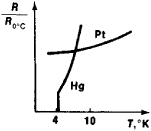Figure 1. Resistance of mercury and platinum as a function of temperature: (R) resistance, (R0°C) value of R at 0°C, (T) temperature, (Hg) mercury, (Pt) platinum. When T = 4.12°K, mercury goes into the super-conductinq state.

Basic phenomena. The sudden disappearance of resistance as the temperature is lowered was first observed by H. Kamerlingh Onnes in mercury in 1911 (Figure 1). Kamerlingh Onnes concluded that at T = 4.15°K the mercury undergoes a transition to a new state, which can be termed superconducting because of its unusual electrical properties. Subsequently, he found that the electrical resistance of mercury is restored when a sufficiently strong magnetic field, called the critical magnetic field Hc, is applied. Measurements have shown that the resistance decreases to zero over a very narrow but finite temperature interval. For pure specimens, the width of this interval is 10-30—10-40K; the width increases when impurities and other structural defects are present.

The lack of resistance in the superconducting state is demonstrated most convincingly by experiments in which a current that is induced in a superconducting ring remains practically unchanged over a period of time. In one version of the experiment, two rings of superconducting metal are used. The larger of the rings is held stationary and the smaller is suspended concentrically by an elastic fiber in such a way that when the fiber is not twisted, the planes of the rings form some angle. The rings are cooled in the presence of a magnetic field below the critical temperature Tc, and the field is then removed. Currents are thereby induced in the rings. The interaction between the currents tends to reduce the initial angle between the planes of the rings. The fiber is twisted, and the observed constancy of the angle of twist shows that the currents in the rings continue to exist. Through experiments of this kind, the resistivity of a metal in the superconducting state has been determined to be less than 10-20 ohm-cm. By comparison, the resistivity of pure copper and silver specimens is about 10-9 ohm-cm at the temperature of liquid helium.

A superconductor, however, is not the simple perfect conductor it was still thought to be more than 20 years after the discovery of superconductivity. The existence of a much deeper difference between the normal and the superconducting states of a metal became apparent when the German physicists W. Meissner and R. Ochsenfeld established in 1933 that a weak magnetic field did not penetrate into the interior of a superconductor. It is particularly significant that this result holds regardless of whether the field is applied before or after the transition of the metal into the superconducting state. By contrast, a perfect conductor—that is, a conductor with a vanishingly small resistivity—should hold a magnetic field that permeates it. This difference is illustrated in Figure 2, where the field distribution in the vicinity of a simply connected metal specimen is depicted schematically for three successive stages of an experiment. In Figure 2,a the specimen is in the normal state, and the external field penetrates freely into the interior of the metal. In Figure 2,b the specimen has been cooled below Tc. The upper drawing shows the magnetic field expelled from the superconductor; for a perfect conductor, as the lower drawing indicates, the field distribution would be unchanged. In Figure 2,c the external field has been removed, and the magnetization of the superconductor has disappeared as well. In the case of an ideal conductor, the flux of the magnetic induction through the specimen would maintain its magnitude, and the field lines would exhibit the same pattern as for a permanent magnet.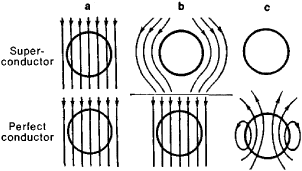Figure 2. Distribution of a magnetic field around a superconducting sphere and around a sphere with a vanishingly small resistance (a perfect conductor): (a) T > Tc; (b) T < Tc, and the external field Hex - 0; (c) T < Tc and Hex 0

The expulsion of the magnetic field from a superconducting specimen is usually called the Meissner effect. This exclusion of the field means that such a specimen in the presence of an external magnetic field acts like an ideal diamagnet of the same shape and with the magnetic susceptibility ξ = – ¼π. In particular, if the specimen is a long solid cylinder and the external field H is uniform and parallel to the axis of the cylinder, then the magnetic moment per unit volume is M = — H/4 π. This value is approximately 105 times larger in absolute magnitude than the specific magnetization of a diamagnetic metal in the normal state. The Meissner effect arises because, when H < HC, there appears in the surface layer of the superconducting cylinder a persistent circulating current of a strength such that the current’s magnetic field cancels the applied field within the interior of the superconductor. Experiment shows that, in the case of large specimens, a weak magnetic field under Meissner-effect conditions penetrates the metal to a depth δ of approximately 10-5—10-6 cm. It is in this layer that the surface current flows.

Depending on their behavior in sufficiently strong fields, superconductors are divided into two large groups: type I and type II. Figures 3 and 4 show in a somewhat idealized form the magnetization curves M (H) that are typical for each group. The curves pertain to the case of long cylindrical specimens placed in a field that is parallel to the axis of the cylinder. In an experiment with this geometry, demagnetization effects are absent, and the situation is therefore much simpler. The initial rectilinear portion of these curves, where M = —H/4π, corresponds to the range of values of H in which the Meissner effect occurs. The two figures show that the subsequent portions of the M (H) curves are very different for type I and type II superconductors.

All the pure superconducting metallic elements, with the exception of V and Nb, are type I superconductors. When the field H = Hc, type I superconductors lose their superconductivity, the field abruptly penetrates the metal, which goes into the normal state throughout its volume. In addition, the specific magnetic moment is suddenly decreased by a factor of approxi mately 105. The critical field Hc can be given a simple thermodynamic interpretation. If the temperature T < Tc and there is no magnetic field, the free energy in the superconducting state Fs is lower than the free energy in the normal state Fn. When the field is applied, the free energy of the superconductor increases by the quantity H2/8π, which is equal to work done during the magnetization. When H = Hc, the free energy becomes equal to Fn (because of the smallness of the magnetic moment in the normal state, Fn is practically unchanged when the field is applied). The field Hc is thus determined from the equilibrium condition at the transition point: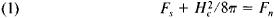The critical field is a function of temperature. The value of the critical field is greatest at T = 0°K and decreases monotoni-cally to zero as Tc is approached. (Values of Hc for some superconductors are given in the article SUPERCONDUCTOR.) Figure 5, a shows the phase diagram in the (H, T)-plane for type 1 superconductors. The crosshatched area under the curve HC (T) corresponds to the superconducting state. All the thermodynamic characteristics of a type I superconductor can be calculated from the measured function HC(T). In particular, an expression for the latent heat evolved in the transition to the superconducting state can be obtained directly from equation (1) by differentiating with respect to temperature: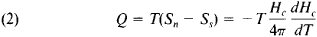where S is the entropy per unit volume. The sign of Q is such that heat is absorbed by the superconductor during the transition to the normal state. Therefore, when the superconductivity of an adiabatically insulated specimen is destroyed by a magnetic field, the specimen is cooled.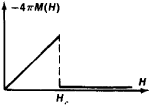Figure 3. Magnetization curve for a type I superconductor

The abrupt nature of the phase transition in a magnetic field (Figure 3) is observed only in the case of a long cylinder in a longitudinal field. For an arbitrary specimen shape and with other field orientations, the transition occurs over a more or less broad interval of values of H. The transition begins when H < Hc and ends when the field exceeds Hc at all points of the specimen. In this interval of values of H, a type I superconductor is in what is known as its intermediate state. The specimen is divided into alternate lamellar regions of the normal and superconducting phases in such a way that the field in a normal region near an interface is parallel to the interface and equal to Hc. As the field is increased, the fraction of the material occupied by normal lamillae increases, and the magnetic moment of the specimen decreases. The configuration of the normal and superconducting regions and the nature of the magnetization curve essentially depend on geometric factors. For example, in a plate that is oriented perpendicularly to the magnetic field, the division into normal and superconducting regions begins in a weak field that is much less than Hc.

The characteristics of the flow of current in a superconductor are closely associated with the superconductor’s magnetic properties. The Meissner effect requires that the currents be a surface current concentrated in a thin layer determined by the depth of penetration of the magnetic field. When the current reaches some critical value sufficient to create a critical magnetic field, a type I superconductor goes into the intermediate state and acquires electrical resistance.

Type II superconductors include most superconducting alloys. In addition, the superconducting metallic elements (type I superconductors) become type II superconductors when a sufficiently large quantity of impurities is introduced into them.

The destruction of superconductivity by a magnetic field is more complicated in nature for type II superconductors. As Figure 4 shows, even in the case of a cylindrical specimen in a longitudinal field the magnetic moment decreases gradually over a considerable range of fields from Hc1, where the field begins penetrating the interior of the specimen, to Hc2, where the complete destruction of the superconducting state occurs. In most cases, a magnetization curve of this type is irreversible (magnetic hysteresis is observed). The magnitude of the hysteresis is very sensitive to the processes used in preparing the specimens. By means of special treatment specimens can sometimes be obtained with a magnetization curve that is almost reversible. The field Hc2 is often extremely high and may reach hundreds of thousands of oersteds (see and ).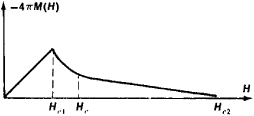Figure 4. Magnetization curve for a type II superconductor

The critical field Hc determined by equation (1) is not a directly observable characteristic for type II superconductors. It can, however, be calculated from experimentally found values of the free energy in the normal and superconducting states in the absence of a magnetic field. The value of Hc calculated by this method lies between Hc1 and Hc2. Thus, the penetration of a magnetic field into a type II superconductor begins in a field less than Hc, where equilibrium condition (1) is still violated in favor of the superconducting state. This seemingly paradoxical phenomenon can be understood if the surface energy of the interfaces between the normal and superconducting regions is taken into account. For type I superconductors, the surface energy is positive; consequently, the appearance of an interface causes an energy loss. This circumstance limits considerably the extent to which a superconductor in the intermediate state is divided up into regions. The anomalous magnetic properties of type II superconductors can be explained qualitatively if the surface energy in this case is taken as negative. The present-day theory of superconductivity leads to precisely this conclusion. A negative surface energy means that the formation of small normal regions oriented along the magnetic field is energetically favorable when H < Hc. The possibility of realizing such a state of a type II superconductor was predicted by A. A. Abri-kosov in 1952 on the basis of the theory of superconductivity advanced by V. L. Ginzburg and L. D. Landau. Abrikosov subsequently made a detailed calculation of the structure of this state. It turned out that the normal regions are produced in the form of filaments that pierce the specimen and have a thickness more or less comparable to the penetration depth of the magnetic field. As the external field is increased, the concentration of the filaments grows, and the magnetic moment consequently undergoes a gradual decrease. Thus, for field values from Hc1 to Hc2, the superconductor is in what is known as the mixed state.

Phase transition to the superconducting state in the absence of a magnetic field. Direct measurements of the specific heat of superconductors when H = 0 indicate that as the temperature is lowered, the specific heat undergoes a jump at the transition point Tc to a value approximately 2.5 times greater than its value in the normal state near Tc (Figure 6). Here, the heat of transition Q = 0—this result follows, in particular, from equation (2), since Hc = 0 when T = Tc. Thus, the phase transition from the normal to the superconducting state in the absence of a magnetic field is a second-order transition. From equation (2) an important equation can be obtained that gives the relation between the jump in the specific heat and the slope of the curve Hc (T) (Figure 5) at the point T = Tc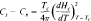where Cs and Cn are the values of the specific heat in the superconducting and normal states, respectively. This equation is in good agreement with experiment.

The nature of superconductivity. The available experimental data on superconductivity demonstrate convincingly that when a conductor is cooled below Tc, it goes into a new state that is qualitatively different from the normal state. While investigating various possible explanations for the properties of a superconductor, especially the Meissner effect, the German scientists H. London and F. London, who were working in England, in 1934 came to the conclusion that the superconducting state is a macroscopic quantum state of a metal. On the basis of this concept they constructed a phenomenological theory that explained the behavior of superconductors in a weak magnetic field—in particular, the Meissner effect and the absence of resistance. In 1950, Ginzburg and Landau introduced a generalization of the London theory that permitted consideration of the behavior of superconductors in strong magnetic fields. The Ginzburg-Landau theory accounted for a large number of experimental data and predicted important new phenomena.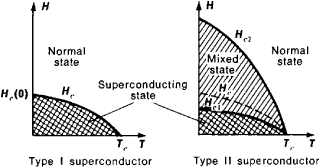Figure 5. Phase diagrams for type I and type II superconductors

A convincing demonstration of the correctness of the London and Ginzburg-Landau theories was provided by the discovery of the quantization of the magnetic flux enclosed within a superconducting ring. It follows from the London equations that the magnetic flux in this case can assume only values that are multiples of the flux quantum Φ0 = hc/e*, where e* is the charge of the supercurrent carriers, h is Planck’s constant, and c is the speed of light. This effect was observed in 1961 by R. Doll and M. Näbauer and, independently, by B. Deaver, Jr., and W. Fairbank of the USA. It was found that e* = 2e, where e is the charge of an electron. The quantization of magnetic flux also occurs in the aforementioned case where a type II superconductor is in a magnetic field stronger than Hcl. The filaments of the normal phase that are formed in this case carry a quantum of flux Φ0.

A consistent microscopic theory of superconductivity was advanced in 1957 by J. Bardeen, L. N. Cooper, and J. R. Schrieffer of the USA and by N. N. Bogoliubov of the USSR. This theory is based on the Cooper effect, confirmation for which was provided by the experimentally found value (e* = 2e) of the charge of the particles that, by their motion, produce a supercurrent. According to Cooper, under certain conditions two electrons having opposite spins can form a bound state, which is called a Cooper pair. The charge of such a pair is equal to 2e. The pairs have a zero spin value and obey Bose-Ein-stein statistics. Cooper pairs are formed when a metal goes into the superconducting state. Since the pairs undergo Bose-Ein-stein condensation (seeQUANTUM FLUID), a system of Cooper pairs has the property of superfluidity. Thus, superconductivity constitutes the superfluidity of the electron fluid. When T = 0°K, all the conduction electrons are bound in pairs. The binding energy of the electrons in a pair is very small— approximately 3.5 kTc, where k is the Boltzmann constant. Excitations arise in the system when pairs break up. A breakup can occur, for example, when a quantum of an electromagnetic field or a sound quantum (a phonon) is absorbed. When the temperature is greater than zero, a certain equilibrium concentration of excitations exists. As the temperature increases, this concentration increases, and the concentration of pairs accordingly decreases. The binding energy of a pair determines a gap in the energy spectrum of the excitations. This energy gap is the minimum energy needed to produce an individual excitation.

In general, it is possible that different kinds of attractive forces between electrons may result in the formation of pairs. In all known superconductors, however, these forces depend on the interaction of electrons with phonons. Nonetheless, the development of the theory of superconductivity has stimulated an intensive theoretical search for other mechanisms of superconductivity. In this regard, particular attention is being devoted to filamentary (one-dimensional) and lamellar (two-dimensional) structures with sufficiently high conductivity. Grounds exist for expecting in such structures a stronger attraction between electrons than in ordinary superconductors. Consequently, such structures can be expected to have a higher critical temperature for transition into the superconducting state.

Phenomena associated with superconductivity apparently occur in some cosmic objects, such as neutron stars.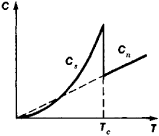Figure 6. Jump in the specific heat of a superconductor at the transition point in the absence of an external magnetic field: (Tc) transition point, (Cs) specific heat in the superconducting state, (Cn) specific heat in the normal state

Practical applications. The practical applications of superconductivity are rapidly expanding. In addition to superconducting magnets and superconducting magnetometers, a number of other devices and measuring instruments exist that are based on various properties of superconductors (see). Superconducting resonant cavities have been built with extremely high (up to 1010) quality factors. Superconducting elements have been developed for computers. Another promising area is the use of superconductors in large electrical machines.

### REFERENCES

De Gennes, P. Sverkhprovodimost’ metallov i splavov. Moscow, 1968. (Translated from English.)
Lynton, E. Sverkhprovodimost’, 2nd ed. Moscow, 1971. (Translated from English.)
Sverkhprovodimost’: Sb. st. Moscow, 1967.
Mendelssohn, K. Na puti k absoliutnomu nuliu. Moscow, 1971. (Translated from English.)
Rose-Innes, A. C, and F. Rhoderick. Vvedenie v fiziku sverkhprovodimosti. Moscow, 1979. (Translated from English.)
Fizicheskii entsiklopedicheskii slovar’, vol. 4. Moscow, 1965. Pages 475–82.

G. M. ELIASHBERG

## superconductivity

[¦sü·pər‚kän‚dək′tiv·əd·ē]
(solid-state physics)
A property of many metals, alloys, and chemical compounds at temperatures near absolute zero by virtue of which their electrical resistivity vanishes and they become strongly diamagnetic.

## superconductivity

Physics the property of certain substances that have no electrical resistance. In metals it occurs at very low temperatures, but higher temperature superconductivity occurs in some ceramic materials
References in periodicals archive ?
Using a laser in a vacuum, the Oak Ridge team vaporized a mixture of powders of the superconductive compound yttrium barium copper oxide (YBCO) and of barium zirconate, which doesn't superconduct. As the vapor condensed on the ribbon, it formed a film of YBCO containing nanometer-scale disks of barium zirconate.
If so, the new finding could shed light on a mechanism that researchers suspect occurs in cuprates, materials of enormous scientific and commercial interest that superconduct electricity at temperatures up to 133 kelvins (SN: 8/15/98, p.
Two years ago, he and his colleagues developed computer models showing that sulfur should turn into a metal at high pressures and superconduct at low temperatures.

Site: Follow: Share:
Open / Close